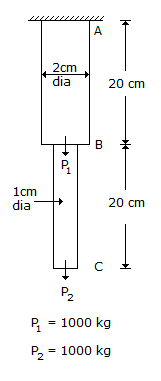# Civil Engineering - UPSC Civil Service Exam Questions

41.

A ball of mass 1 kg moving with a velocity 2 m/s collides directly with a m2 stationary ball of mass 2 kg. If the first ball comes to rest after the impact, what is the velocity of the second ball after impact ?

 A. 1.0 m/s B. zero C. 2 m/s D. 0.5 m/s

Explanation:

No answer description available for this question. Let us discuss.

42.

What is number of categories into which masonry buildings are divided on the basis of earthquake resistance features ?

 A. Five B. Four C. Three D. Two

Explanation:

No answer description available for this question. Let us discuss.

43.

A mild steel bar is 40 cm long. The lengths of parts AB and BC of the bar are 20 cm each. It is loaded as shown in the given figure. The ratio of the stresses, σ1 in part AB to σ2 in part BC isA. 2 B. 1/2 C. 4 D. 1/4

Explanation:

No answer description available for this question. Let us discuss.

44.

Zero hardness of water is achieved by :

 A. using lime soda process B. excess lime treatment C. ion exchange method D. using excess alum dosage

Explanation:

No answer description available for this question. Let us discuss.

45.

The normal duration and normal cost of an activity are 10 days and Rs 350/- respectively. The cost slope is Rs 75/- per day. If the crash duration is 8 days, then what is the crash cost of the activity ?

 A. Rs 400/- B. Rs 500/- C. Rs 600/- D. None of these